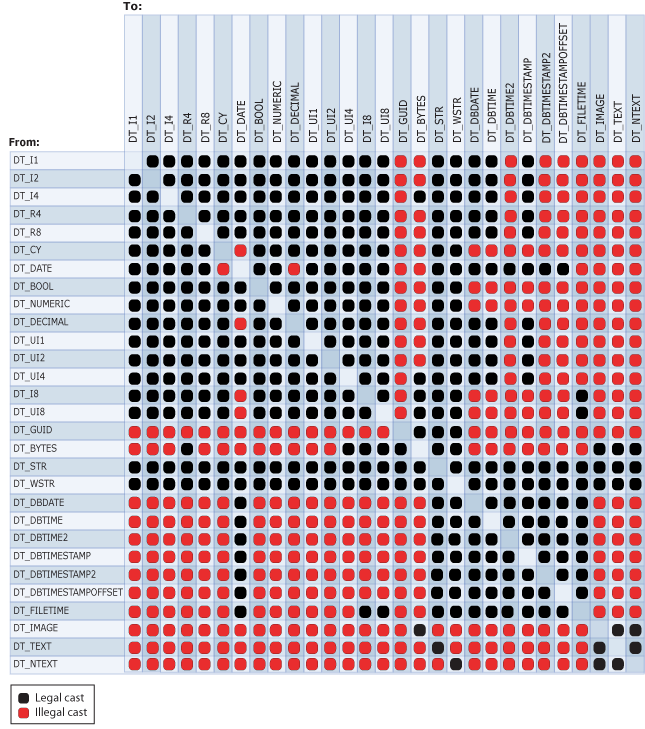# Cast (SSIS Expression)

Applies to:SQL Server (all supported versions)SSIS Integration Runtime in Azure Data Factory

Explicitly converts an expression from one data type to a different data type. The cast operator can also function as a truncation operator.

## Syntax

``````
(type_spec) expression

``````

## Arguments

type_spec
Is a valid SSIS data type.

expression
Is a valid expression.

## Result Types

The data type of type_spec. For more information, see Integration Services Data Types.

## Remarks

The following diagram shows legal cast operations.Casting to some data types requires parameters. The following table lists these data types and their parameters.

Data type Parameter Example
DT_STR charcount

codepage
(DT_STR,30,1252) casts 30 bytes, or 30 single characters, to the DT_STR data type using the 1252 code page.
DT_WSTR Charcount (DT_WSTR,20) casts 20 byte pairs, or 20 Unicode characters, to the DT_WSTR data type.
DT_BYTES Bytecount (DT_BYTES,50) casts 50 bytes to the DT_BYTES data type.
DT_DECIMAL Scale (DT_DECIMAL,2) casts a numeric value to the DT_DECIMAL data type using a scale of 2.
DT_NUMERIC Precision

Scale
(DT_NUMERIC,10,3) casts a numeric value to the DT_NUMERIC data type using a precision of 10 and a scale of 3.
DT_TEXT Codepage (DT_TEXT,1252) casts a value to the DT_TEXT data type using the 1252 code page.

When a string is cast to a DT_DATE, or vice versa, the locale of the transformation is used. However, the date is in the ISO format of YYYY-MM-DD, regardless of whether the locale preference uses the ISO format.

Note

To convert a string to a date data type other than DT_DATE, see Integration Services Data Types.

If the code page is a multibyte character code page, the number of bytes and characters may differ. Casting from a DT_WSTR to a DT_STR with the same charcount value may cause truncation of the final characters in the converted string. If sufficient storage is available in the column of the destination table, set the value of the charcount parameter to reflect the number of bytes that the multibyte code page requires. For example, if you cast character data to a DT_STR data type using the 936 code page, you should set charcount to a value up to two times greater than the number of characters that you expect the data to contain; if you cast character data using the UTF-8 code page, you should set charcount to a value up to four times greater.

For more information about the structure of date data types, see Integration Services Data Types.

## SSIS Expression Examples

This example casts a numeric value to an integer.

``````(DT_I4) 3.57
``````

This example casts an integer to a character string using the 1252 code page.

``````(DT_STR,1,1252)5
``````

This example casts a three-character string to double-byte characters.

``````(DT_WSTR,3)"Cat"
``````

This example casts an integer to a decimal with a scale of two.

``````(DT_DECIMAl,2)500
``````

This example casts an integer to a numeric with a precision of seven and scale of three.

``````(DT_NUMERIC,7,3)4000
``````

This example casts values in the FirstName column, defined with an nvarchar data type and a length of 50, to a character string using the 1252 code page.

``````(DT_STR,50,1252)FirstName
``````

This example casts values in the DateFirstPurchase column of type DT_DBDATE, to a Unicode character string with a length of 20.

``````(DT_WSTR,20)DateFirstPurchase
``````

This example casts the string literal "True" to a Boolean.

``````(DT_BOOL)"True"
``````

This example casts a string literal to DT_DBDATE.

``````(DT_DBDATE) "1999-10-11"
``````

This example casts a string literal to the DT_DBTIME2 data type that uses 5 digits for fractional seconds. (The DT_DBTIME2 data type can have between 0 and 7 digits specified for fractional seconds.)

``````(DT_DBTIME2, 5) "16:34:52.12345"
``````

This example casts a string literal to the DT_DBTIMESTAMP2 data type that uses 4 digits for fractional seconds. (The DT_DBTIMESTAMP2 data type can have between 0 and 7 digits specified for fractional seconds.)

``````(DT_DBTIMESTAMP2, 4) "1999-10-11 16:34:52.1234"
``````

This example casts a string literal to the DT_DBTIMESTAMPOFFSET data type that uses 7 digits for fractional seconds. (The DT_DBTIMESTAMPOFFSET data typecan have between 0 and 7 digits specified for fractional seconds.)

``````(DT_DBTIMESTAMPOFFSET, 7) "1999-10-11 16:34:52.1234567 + 5:35"
``````